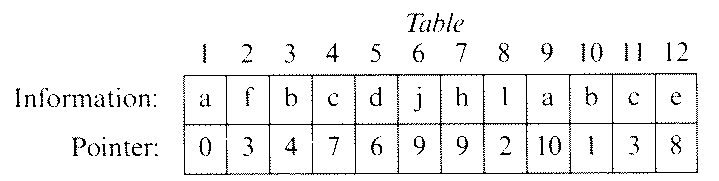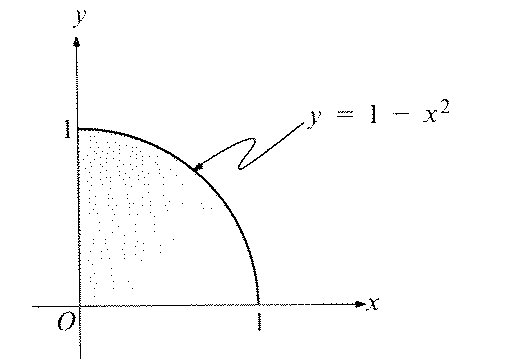1988 APCS B Multiple Choice

This page contains the 15 B multiple choice questions from the 1998 Advanced Placement Exam in Computer Science. Not all formatting has been reproduced (particularly in code fragments). If you believe there is an error in the text, please report it to Stuart Reges. See the copyright notice at the end of this document.

1. Suppose that Table is an array of records used to implement a linked list, as shown below.Each component of Table contains an information field and a pointer to the next element in a linked list. (The pointer values are indices of Table, and 0 signifies the end of a list.) What is the list off characters starting with Table?

1. da
2. djaba

2. Which of the following is true of stacks and queues?
1. A stack is a last-in, first-out structure, and a queue is a first-in, first-out structure
2. A stack is a first-in, first-out structure, and both structures are random access structures.
3. A stack is a last-in, first-out structure, and a queue is a random access structure.
4. A queue is a last-in, first-out structure, and a stack is a first-in, first-out structure.
5. A queue is a first-in, first-out structure, and a stack is a random access structure.

Questions 38-39 are based on the following information.

A newspaper route has recently been computerized. Information about each of the 100 customers is stored in individual records containing first name, last name, and payment due. In writing a computer program to process the customer records, the programmer is uncertain whether to add a procedure to sort the records.

3. If the records are not sorted, what is the maximum possible number of comparisons that must be made to obtain a particular customer's record using a sequential search?
1. 100
2. 50
3. 7
4. 5
5. 3

4. If the records are first sorted, what will be the maximum number of comparisons needed with a binary search to find a particular customer's record?
1. 100
2. 50
3. 7
4. 5
5. 3

5. What is the least order of execution time for the following code segment, if the segment terminates without error?
i := 1;
Count := 0;
while i < N do
begin
Current := A[i];
while (Current = A[i]) and (i < N) do
i := i + 1;
Count = Count + 1
end

1. O(log N)
2. O(√N)
3. O(N)
4. O(N log N)
5. O(N2)

6. If the inorder traversal of the binary tree T is
A D B G C F E
and each node of T has either 0 or 2 children, which of the following nodes is NOT a leaf of that tree?
1. A
2. B
3. C
4. D
5. E

7. In a procedure, value parameters do not necessarily protect the contents of the caller's data structures from being affected by execution of the procedure under which of the following conditions?
1. The procedure is recursive.
2. The value parameters are integers.
3. The value parameters are pointers.
4. The value parameters are arrays.
5. The procedure is used with a forward declaration.

8. The alpha-mem, a new data structure, supports two operations.

The insert operation allows "words" to be stored in the alpha-mem.

The remove operation causes the "word" in the alpha-mem which is first alphabetically to be printed and removed from the alpha-mem.

Which of the following is true of an alpha-mem?

1. If words are inserted in alphabetical order and all words are inserted before any are removed, then it works like a stack
2. If words are inserted in alphabetical order, then it works like a stack whether or not all inserts precede any removes.
3. If all words are inserted before any are removed, then it works like a queue.
4. If words are inserted in reverse alphabetical order, then it works like a queue whether or not all inserts precede any removes.
5. If words are inserted in alphabetical order, then it works like a queue whether or not all inserts precede any removes.

9. Consider the partially completed program below.
Root := 0;
Lim := n;
while BBB do
{ Invariant:  (Root)2 ≤ n ≤ (Lim + 1)2 }
begin
<code to increment Root or decrement Lim,>
<leaving Invariant true                  >
end

With which of the following should BBB be replaced in order for the loop above to compute an integer approximation of the square root of non-negative n>?
1. Lim <> Root
2. Lim = Root
3. Root * Root <> n
4. Lim * Lim <> n
5. Lim * Lim = Root * Root

10. Suppose that the only Pascal implementation available does not support pointers or dynamic storage allocation using new. A Pascal program is given that uses pointers and new for creating and manipulating instances of the following type.
type
NodePtr = ^Node;
Node = record
Info : integer;
Next : NodePtr
end;

To modify this program so that it can be run using the available resources, one should
1. simulate pointers by using indices into a large array, and change the program accordingly
2. simulate pointers by using trees and recursion
3. simulate pointers by using a combination of value parameters and var parameters
4. modify the Pascal implementation so that it does support pointers and new
5. identify the queues and stacks in the program, and reimplement each by using an array

11. A property of binary trees is said to be an "inherited" property if each of the subtrees has the property whenever the binary tree itself does. One inherited property for binary tree is having
1. an odd number of nodes
2. an even number of nodes
3. the same number of nodes in the left and right subtrees
4. at least 10 nodes
5. no node with exactly one child

12. Consider the following procedure.
procedure Delete(p : Pointer);
{ Deletes the node pointed to by p from a doubly linked list }
{ (without a header node) whose pointer fields are called    }
begin
end;

In which of the following cases will the procedure above fail to work properly?
1. p points to the first node in the list.
2. p points to the last node in the list.
3. p = nil

1. III only
2. I and II only
3. I and III only
4. II and III only
5. I, II, and III

13. Of the following, which has the most impact on the efficiency of searching for an item in a hash table?
1. The number of nonkey fields
2. The size of the table
3. The density of the table
4. Whether the size of the table is a prime number
5. The difficulty of computing the inverse of the hash function

14. Of the following data structures, which would be best suited for an application where search time must be minimized and where data can be easily added?
2. Sorted array
3. Hash table
4. Sequential file15. The program segment below uses a Monte Carlo technique to approximate the area of the shaded region shown. The function Random returns a real number in the range 0 ≤ Random < 1 each time it is executed. Assume that the numbers are randomly chosen.
Points := 0;
for i := 1 to n do
begin
x := Random;
y := Random;
S
end;
Area := Points/n

Which of the following statements should be used in place of S to make the segment work as indicated?
1.   if y >= 1 - x*x then Points := Points + 1

2.   if y <= 1 - x*x then Points := Points + 1

3.   if y < 1 - x*x then
Points := Points + 1
else
Points := Points - 1

4.   if y = 1 - x*x then Points := Points + 1

5.   if y > 1 - x*x then Points := Points + 1

The contents of this web page come from The 1988 Advanced Placement Examinations in Computer Science and Their Grading. The following copyright notice appears in that booklet and applies to the content of this page: